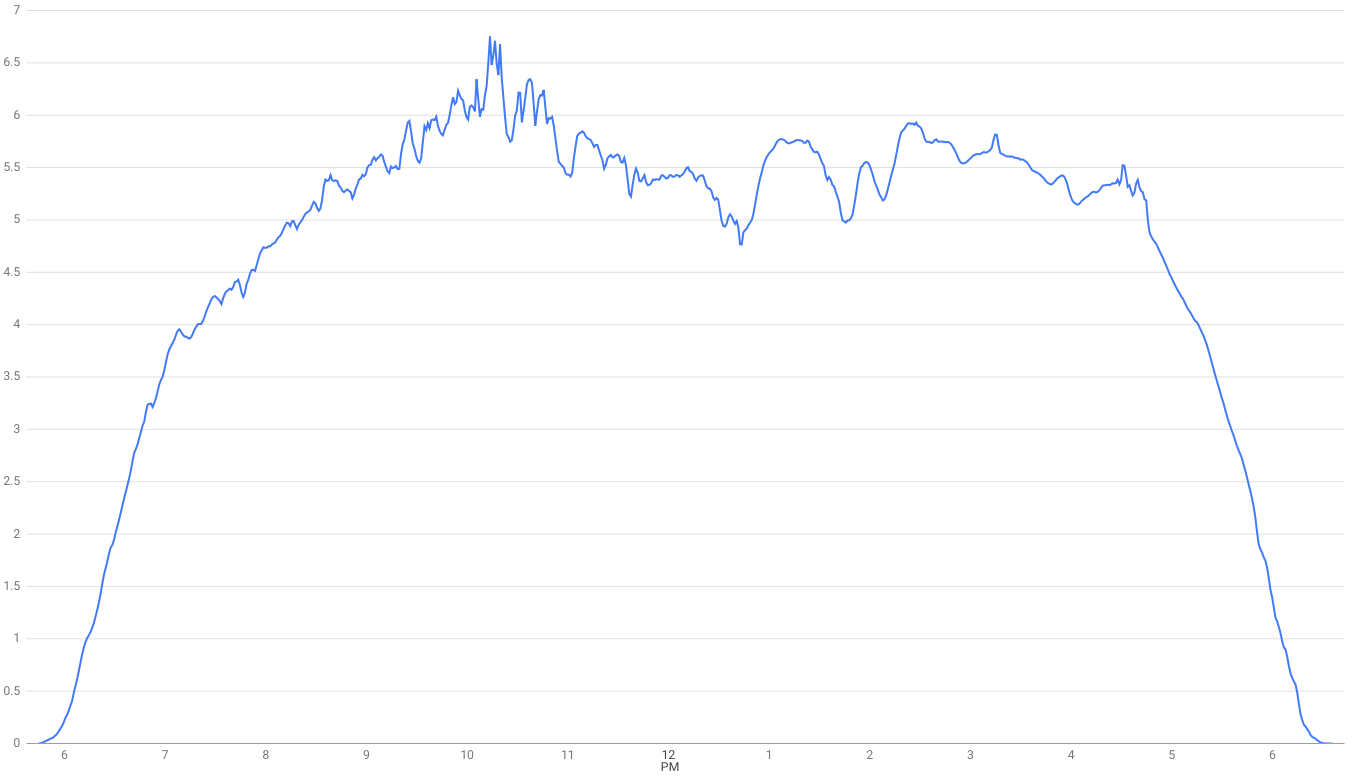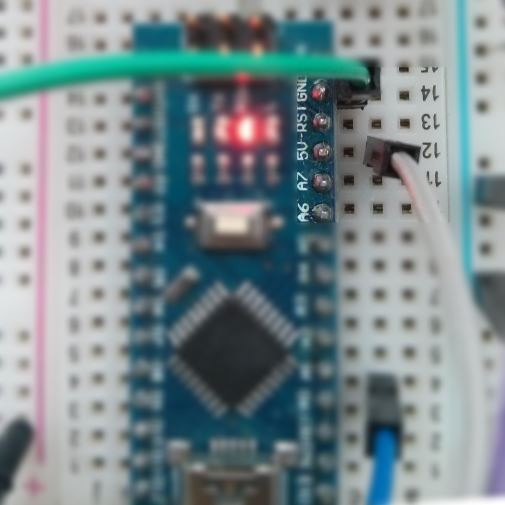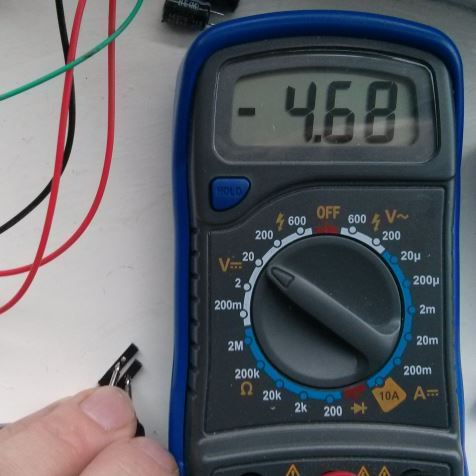I'm including the Arduino source code for a logging analog sensor values. It will capture a reading every second and print it to the Serial output, this should then be monitored by a PC or Raspberrry PI.Sunday's voltage response from my solar panel

##### Calibrating power supply

The Arduino code makes use of an Analog to Digital Converter or ADC which has a 10-bit resolution. This means that when reading the voltage from an analog pin we will get a result between 0 and 1023 where 0 represents 0v and 1023 would represent 5v. This assumes that the power supply is providing a clean and constant 5v and when powering the Arduino via USB this is often not the case.
A miscalibrated value would still provide a useful value for tracking voltage response from a solar panel, but it could be misleading when an analog temperature sensor is involved.I plugged in two male to female jumper wires, one on 5v and one on GND (make sure you don't connect these together) and then use a multi-meter to read the value.#### Arduino source-code

``````void setup() {
Serial.begin(9600);
pinMode(A0, INPUT);
}

void vMeasure(int pin) {
float vout=0.0;
float vin=0.0;
float r1=10000;
float r2=10000;
// float ref = 5.0;
float ref = 4.68;
vout = ((float)value * ref) / 1023.0;
vin = vout / (r2 / (r1+r2));

Serial.print("V(");
Serial.print(pin);
Serial.print("): ");
Serial.print(vout);
Serial.print(" - ");
Serial.println(vin);
}

void loop() {
vMeasure(A0);
delay(1000);
}
``````

The Arduino will only safely measure up to 5v through its ADC, so I have used a resistor divider with two resistors of equal impedance meaning the voltage measured can range up to 10v. r1 and r2 are both `10k` resistors, so if you want to try the code - replace the values with the resistors you have at hand.
You will also see that the value of 'ref' corresponds to the multi-meter reading.

Measuring over 10v?

Vin = Vout * r2 / (r1 + r2)

If your panel or battery can produce a voltage greater than 10v then you can still use a resistor divider, but a different ratio will be needed between r1 and r2.

### Subscribe to Alex Ellis' Blog

Subscribe to keep in touch. By providing your email, you agree to receive marketing emails from OpenFaaS Ltd